# Community

Blog Events Webinars Tutorials Forum
×
Community Blog About Database Kernel | How Does PolarDB HTAP Implement IMCI Query Optimization?

# About Database Kernel | How Does PolarDB HTAP Implement IMCI Query Optimization?

This article uses the join reorder of Polar DB MySQL In-Memory Column Index (IMCI) to describe the query optimization process of PolarDB HTAP.

By Yukun Liang (Responsible for the Research and Development of PolarDB In-Memory Column Index Optimizer)

## 1. Role and Principle of Query Optimization

During a database query, the optimizer accepts the query inputs by a user, performs a series of equivalent transformations on the query, and selects the optimal plan from the equivalent execution plans by estimating the cardinality and cost in the query. Since the execution plan has a great impact on performance, the query optimizer is a critical component in all database systems. The following figure shows a typical query optimizer framework: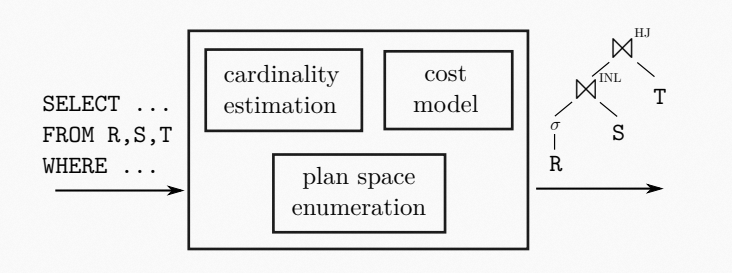Generally, a query optimizer uses the following three typical components to complete query optimization:

• Plan Space Enumeration: It generates multiple execution plans equivalent to the query based on a series of equivalent transformation rules.
• Cardinality Estimation: It estimates the amount of data/data distribution during querying based on the distribution of query tables.
• Cost Model: It computes the cost required for each execution based on execution plans and the internal status of the database.

Among the problems related to query optimization, the most widely studied is the join order of query plans. Since join order can affect query performance, and it depends on the capabilities of the three components above at the same time, it is not too much to say it is the most critical problem in query optimization. This article uses the join reorder of Polar DB MySQL In-Memory Column Index (IMCI) to describe the query optimization process of PolarDB HTAP.

Different database systems use their unique methods to solve join order problems. Although the general framework is shown in the figure above, the specific implementations are different. They can be divided into the following two types:

### 1.1 Bottom-Up Optimization: PostgreSQL & MySQL

PostgreSQL and MySQL use a kind of bottom-up optimizer that divides query optimization into the following three steps:

• Simple Logical Transformation and Preprocessing: At this stage, some operations that are always profitable are tried. For example, MySQL converts outer join to inner join, prunes partitions, and deduces equivalent values. PostgreSQL optimizes constant expressions, expands predicates, and pulls subqueries. Since this part of the operation always has an optimization effect, it is better to deal with it as early as possible to reduce the complexity of the subsequent cost-based query optimization.
• Cost-Based Join Reorder: In this phase, the optimizer enumerates a large number of candidate join plans and computes the cost with the help of the cardinality estimation and cost model to obtain the best join order. PostgreSQL uses a DPsize algorithm with System-R style, while MySQL uses a greedy + enumeration algorithm.
• Optimization after Join: After the join order is determined through optimization, the query optimizer continues to optimize other parts, such as group by, order by, and distinct.

Such a framework is similar to a System-R optimizer. It has the advantage that the search process is intuitive and easy to understand. At the same time, the width of the search is limited by the way of splitting problems, which can ensure that optimization is less time-consuming. However, there are some problems with such a framework.

• Splitting problems is a greedy algorithm that combines the optimal solution of each part. There may be a possibility of falling into local optimization, and some complex query optimizations may not be applied to such a framework.
• Pruning is limited. Some costly query plans may likely be generated during optimization, and these query plans could have been pruned out in advance.
• The optimization process is clear, but it is difficult to expand because there is no unified framework.

### 1.2 Top-Down Optimization: Greenplum, SQLServer, and PolarDB-X

Different from bottom-up optimization, top-down query optimization adopts the idea of dynamic programming. This kind of optimizer adopts the framework of cascade optimizer. The advantage is that query transformation, cost estimation, and pruning are combined, and the whole query optimization is regarded as a whole. The optimization process is to continuously generate new equivalent execution plan fragments through rules transformation and prune after computing the generated execution plans. This framework overcomes the shortcomings of bottom-up optimization and makes optimizer development and expansion more convenient. In recent years, newly developed analytical database systems have adopted this framework. Please refer to  for more details.

## 2. New Challenges Brought by HTAP to Optimizers

PolarDB for MySQL is initially oriented to OLTP scenarios. The core goal of its optimizer is to achieve optimal performance under some simple or fixed-pattern queries. PolarDB for MySQL optimizer performs a large number of optimizations related to its execution model to achieve this (such as executing and eliminating subqueries in the query optimization phase and eliminating order by clauses based on index order). These optimizations have a good effect on query execution but make PolarDB for MySQL optimizer bound to its execution model and storage format.

However, under HTAP load, many complex queries need to be accelerated by row storage + column index (replica) + execution layer optimized for column storage. Under such conditions, a large number of design assumptions in the original PolarDB for MySQL optimizer are broken. At the same time, due to the coupling of the original optimizer with execution models and storage, simple modifications can hardly make the optimizer adapt to query optimization capabilities that HTAP loads. For databases that have to deal with different storage, execution models, and different data models, an optimizer that supports HTAP load scenarios should do the following:

• Avoid tight coupling with the storage/execution layers and adapt to row-format/column-format storage simultaneously and facilitate future functional evolution
• Compare cost before row/column indexes and select the optimal plan. That means, in addition to the original row-based optimization mechanism, the optimizer can handle multi-dimensional filtering, joining, and aggregation of complex queries based on column store.

## 3. Query Optimization of PolarDB IMCI

### 3.1 Optimization of Row-Based Plans and the Limits

The native optimizer of PolarDB for MySQL has a clear optimization process. The query optimization process is listed below:

1.  Apply some rule-based optimization. The rules here usually make plans better. Therefore, cost computing is not involved here. For example:

a) Convert partial outer join to inner join

b) Equivalent Derivation: `c1 = 5 and c1 = c2` can be converted to `c1 = 5 and c2 = 5`.

c) Partition Pruning: If the query only falls on some partitions, other partitions will not be opened, which can reduce the amount of data scanned.

d) Subquery Elimination: Some subqueries that only return one row will be executed in advance and replaced with the results, which can simplify plans.

2.  Cost-Based Join Reorder: As mentioned above, compute cost through the greedy + enumeration algorithm and available indexes.

3.  Follow-Up Optimization: Determine the access method of the table and optimize the order by and distinct based on the indexes used

The optimization process is clear and good enough in the row-based iterator execution mode. However, after PolarDB adds a column index, this optimization system exposes the following problems:

• Join Reorder can only generate the execution plans of the left deep tree. The optimal execution plan may be missed in complex queries in OLAP scenarios. The rewriting of SQL statements through subquery + join order hint may change the business significantly.
• When enumerating execution plans, the number of input rows for each join and the selection rate are computed. In the design of the row-based execution layer, IndexJoin is the best execution mode for join. For this reason, the optimizer relies on creating secondary indexes on tables to estimate the selection rate when computing the cost. If there is no secondary index, the cost estimation error may be large. This design is consistent with row-based scenarios: join is completed using indexes, and slow queries are resolved by adding indexes. However, when facing commonly seen multi-dimensional filtering, join, and aggregation operations in complex analysis scenarios, it is inappropriate to add a large number of secondary indexes because adding indexes means equally spreading read load to write operations. As a result, a large number of secondary indexes occupy a large amount of storage space and slow down the write efficiency of the database.
• Implementing Join Reorder and other optimizations separately can improve search efficiency but may fall into local optimization.

### 3.2 Optimization Process of IMCI Plans

PolarDB IMCI adds a new optimization process to the original optimizer framework to solve the performance defects of the native optimizer under HTAP workload. This optimizes the original optimizer framework to meet the requirements of the original row-based OLTP, complex queries, column storage formats, and vectorized parallel execution operators. On the whole, we use rule-based rewriting combined with cost-based query optimization to obtain better execution plans.

#### 3.2.1 Rule-Based Query Plan Rewriting

In addition to the existing rules of the PolarDB for MySQL optimizer, we have added some new rules. These rules have two main functions: make execution plans meet the requirements of the vectorized executor and optimize the efficiency of the column executor to execute queries. For example:

• Disassociate Subqueries: In the absence of indexes, the execution of associated subqueries is similar to nested loop join, which leads to poor execution efficiency. IMCI converts associated subqueries into joins through subquery disassociation technology and uses hash join to efficiently execute associated subqueries.
• Remove aggregate functions that contain DISTINCT from subqueries. For example:
``````SELECT COUNT(DISTINCT c1) FROM t;
-- will be converted to
SELECT COUNT(c1) FROM (SELECT c1 FROM t GROUP BY c1);``````

Through this conversion, the design of the column executor can be simplified, and more functions can be supported with a streamlined implementation.

• Reorder Predicates: In the case of multiple predicates existing in a single-table scan, such as `t1.c2 LIKE '%cat%' AND t1.c1 = 5`, because of the lower execution cost and selection rate of `t1.c1 = 5`, executing `t1.c1 = 5` first can significantly reduce the heavier `LIKE` operations and improve the execution efficiency of queries.

This step also includes some rules that should be based on cost evaluation but can make queries better in most cases, such as predicate pushdown. By applying these rules in this step, even though the subsequent optimization and execution layers get standardized input, the advance of predicate pushdown operations reduces the cost-based query optimization space and improves efficiency.

#### 3.2.2 Cost-Based Query Optimization

After the rule-based query plan rewriting process, the query plan is optimized through cost-based optimization. In IMCI, we use a cascade-like optimizer framework for query optimization, which can help avoid the local optimization problems that may exist in query optimization. In this framework, we use the three functional modules of the traditional optimizer: plan enumeration, cardinality estimation, and cost model. Here, we take the join order problem as an example to describe the internal details and workflow of each module.

##### 3.2.2.1 Plan Enumeration

This is the component used by the optimizer to enumerate plans. After a user's SQL statement undergoes a series of steps (such as parse and binding), an initial query plan is generated and input to the optimizer. The optimizer performs various equivalent transformations on the input query plan through rules to generate new equivalent query plans. In IMCI, these rules used to rewrite plans are divided into the following two categories:

• Transform Rule: These rules are similar to the rewrite in the previous step, but they are not as widely used as the former. Blind rewrite by these rules may lead to a decrease in query performance. Therefore, such rules are put into the IMCI cost-based optimizer to enumerate equivalence query plans. Later, the cost of queries is computed to determine whether to use these rules.
• Implement Rule: This kind of rule is used to choose between logical plans and physical plans, which is often called algorithm selection. For example, for join, we can choose hash join, index join, sort merge join, etc. Since each algorithm has advantages and disadvantages in different scenarios, this kind of rule is also put into IMCI cost-based optimizer.

In this module, the execution plan will generate a large number of equivalent plans through these rewriting rules, and the best execution plan will be selected through cost comparison. IMCI uses the cascade optimizer framework, which saves common intermediate results through data structures (such as Memo, Group, and GroupExpr), reducing a large number of redundant intermediate results. However, queries containing a large number of joins may still generate a large number of equivalent plans. For a join formed by n tables, there are at least 2 ^ n possible join orders. Without considering the Cartesian product, the relationship between the number of join plans and the enumeration time is shown in the following figure: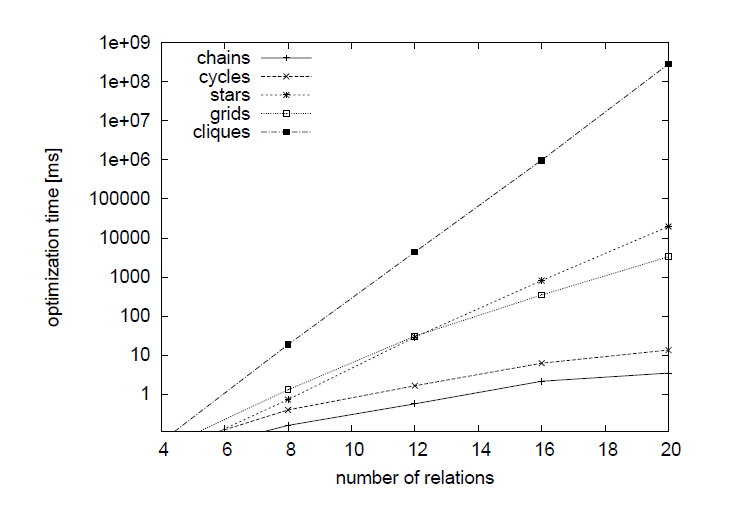In the worst case, the optimization time of a join reorder containing 12 tables exceeds ten seconds. For common star queries, the optimization time exceeds ten seconds for 20 tables. This may be unacceptable for some queries containing a large number of tables. Therefore, when we want to select the best join order, we must cope with the following three problems:

1. The join plans enumerated contain the best plan. In order to achieve this goal, the enumeration efficiency must be high enough, so the best join plan can be included in the search space as much as possible.
2. If there are too many tables to join, a stable heuristic algorithm can search for a good query plan in an acceptable time.
3. Among all the listed join plans, choose the best plan through cardinality estimation and cost model.

The method of each system is different for the enumeration of join order. PostgreSQL uses an algorithm based on dynamic programming enumeration + heuristic algorithms. When the number of tables is small (<12), PostgreSQL uses a DPsize algorithm based on dynamic programming ideas. When the number of tables is large, the genetic algorithm (GEQO) is used as a heuristic algorithm to compute the join order. Greenplum is the same as the PostgreSQL algorithm when the number of tables is small. When the number of tables is large, it abandons GEQO and uses a greedy algorithm as the heuristic algorithm. MySQL uses an exhaustive + greedy algorithm. Due to the poor efficiency of the exhaustive algorithm, the enumeration depth is controlled by the parameter `optimize_search_depth = k`. Each time, MySQL enumerates the k table join with the smallest cost and determines the prefix of the current join according to the result (the greedy idea is used here). This process is repeated until the number of remaining tables is less than k, and the join order of the remaining k tables is determined by the last exhaustive.

In the enumeration phase of IMCI, we mainly solve problems 1 and 2 above. For problem 1, IMCI currently adopts the same DPhyp algorithm as DuckDB and Hyper. Compared with the DPsize algorithm used by GPORCA and PostgreSQL, this algorithm has the main advantage that it is more efficient. DPsize algorithm is an enum-and-test algorithm, which can generate a large number of invalid plans during enumeration. In contrast, the DPhyp algorithm constructs a graph according to join conditions and enumerates plans according to the graph. This decreases invalid enumerations and improves. For different kinds of joins of n tables, the complexity of the algorithm used for join reorder in each system is listed below: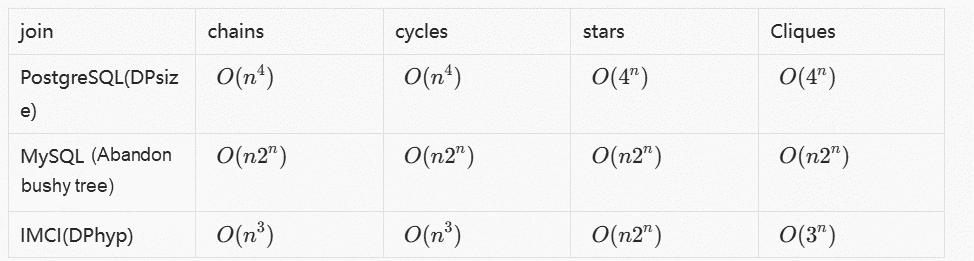As shown in the table, the complexity of the DPhyp algorithm is optimal among the algorithms that do not limit the enumeration plan shape (MySQL only generates the left deep tree). The following figure shows the efficiency comparison between DPhyp and DPsize enumeration: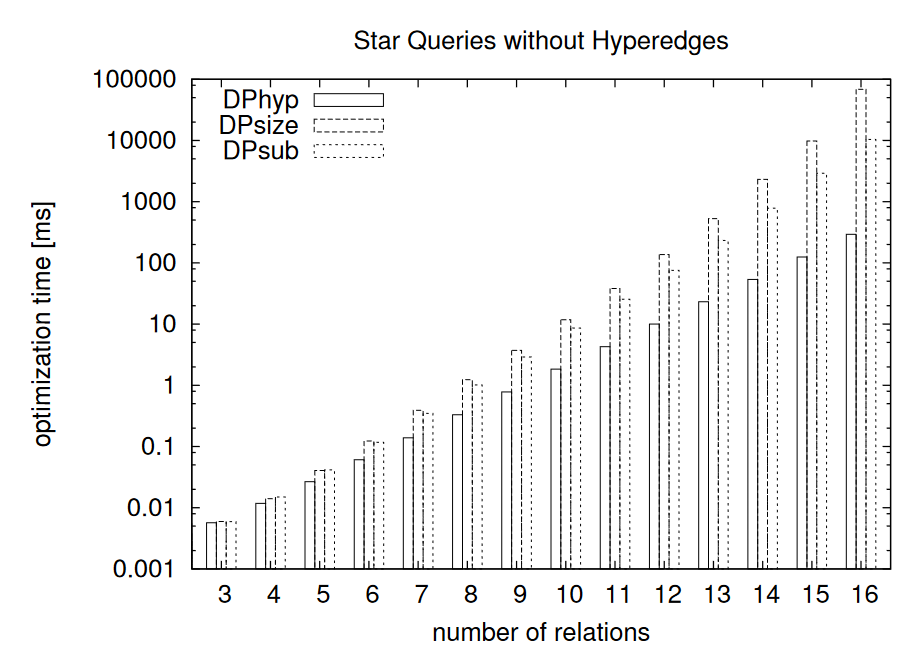For problem 2, currently, we retain the join order generated by the row-based optimizer (which means the join plans of its own are regarded as the product of the heuristic algorithm). In the case of a large number of tables, IMCI only enumerates some join plans. On this basis, we add the join order generated by the native optimizer (equivalent to partially extending the join plans through the DP algorithm) and select the best plan from them. In addition, by incorporating the DPhyp algorithm into the cascade optimizer, we use the cascade optimizer framework to avoid local optimization that may occur due to separate consideration of joins.

##### 3.2.2.2 Cardinality Estimation and Cost Model

In the enumeration section above, the query optimizer enumerates a large number of plans. The next step is to compute the cost of each equivalent query plan and select the best execution plan from it. The existing optimizers usually follow the following two steps to achieve this:

• Estimate the approximate distribution of the number of input/output rows of each operator and the output data of this operator through cardinality estimation
• Compute the cost through the cost model, add the execution cost of each operator to obtain the total cost, and select the execution plan with the lowest total cost.

Cardinality computing depends on two modules: the collection and computing of statistics and the logic of computing the number of input and output rows.

Collection of Statistics

IMCI has developed a statistics module for the new optimizer to optimize queries in the absence of sufficient secondary indexes. IMCI estimates the number of output/output rows of operators by collecting the following information from the table.

• The cardinality of each column (the number of unique values on the column, which is equivalent to `COUNT(DISTINCT col)`)
• The proportion of NULL values in each column
• Maximum and minimum values for each column
• Histogram created on each column based on values
• Unique key and foreign key attributes on the table

Construction of Statistics

In order to collect these statistics, we compute the number of rows to be sampled based on the amount of data in the table. The number of rows to be sampled is determined by the following formula given in :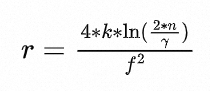Where n is the size of the table, k is the number of buckets in the histogram, the confidence interval of relative error is f, and the confidence level is γ. After the optimizer computes the number of sampled rows based on the appropriate constants, we build a histogram using the input rows to compute the proportion of NULL values. Then, the optimizer computes the cardinality of the column based on the sampled data, which is related to the sampling method. There are many formulas available for the estimation of the cardinality on the column, each of which makes different assumptions based on the data. For example, in the following formulas, we make D as the estimated result, n as the number of sampled rows, q as the number of sample rates, fi as the number of values that happen to occur i times in the sample, and d as the cardinality of the sample.

•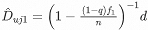This is the formula used to estimate the distinct value of a column in PostgreSQL. This formula assumes the data is evenly distributed, so it is often used when the sample rate is small.
•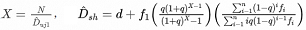This formula assumes the distribution of sampled data and the real data is the same, so the formula is often used when the sample rate is large (usually 10% or more).
•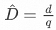It is applicable when the sample rate is large.

In IMCI, we compute the sample rate based on the number of sampled rows and select the appropriate computing formula to compute the number of distinct values based on the size of the sample rate, keeping the error as minimal as possible.

Another problem is that these formulas usually require uniform random sampling. However, in column storage, data is usually not stored in pages. In IMCI, 64K rows are usually compressed and stored together. Then, to read a row, we need to read the entire data block corresponding to the row from the disk and decompress it. The read amplification problem is serious.

In order to solve this problem, we choose to sample according to data blocks to reduce read amplification and use the COLLAPSE algorithm mentioned in  to correct the computing formula of distinct values. There is a general idea. If multiple identical values are appearing in the same block, they would be computed as appearing only once because the estimation formula above largely depends on f1. This approach corrects the estimation error based on uniform random sampling by reducing the impact of data locality.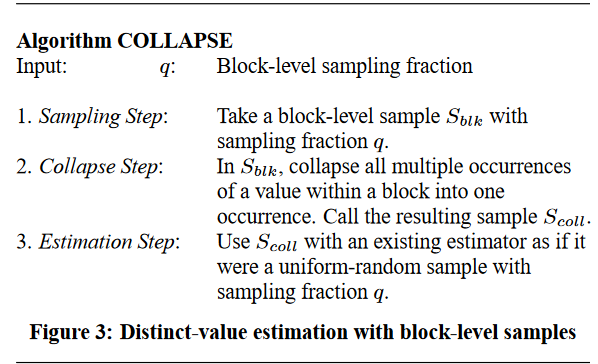How to Make Number Estimation

The only operators that need to use statistical information to estimate the number of rows are filter, join, and groupby. The output of other operators is usually only related to the number of input rows (such as sorting). Through histograms, we can effectively estimate the selection rate of predicates like `a > 10 AND a < 100`, and we can compute the selection rate of equal join and range join through histogram join. The cardinality on the column can help us estimate the number of groups output by group by and assist us in estimating the selection rate of equivalent predicates and equal join when some histograms are unavailable, such as`Selectivity(a = 1) = 1 / COUNT(DISTINCT a)`. Unique key and foreign key can help us correct the results when computing equal join, such as the following SQL:

``````SELECT COUNT(*)
FROM t1, t2
WHERE t1.a = t2.a;``````

When t1.a is the unique key on t1 and t1.a is the foreign key of t2.a, we can get additional information: for each row of t2, there must be a row in t1 that matches. Therefore, we can accurately get the number of output rows of this join and the size of the t2 table.

Selection Rate Computing of Multi-Predicate Combination

In PostgreSQL, if a predicate is composed of conditions of multiple columns:

``````-PAY TIME for Beijing Time

SELECT COUNT(*)
FROM PAY_INFO
WHERE NATION = 'CHINA' AND
PAY_TIME BETWEEN '2008-12-20 18:00:00' AND '2008-12-20 21:00:00'``````

Let’s assume the table stores the payment data of users in China, Germany, and the United States, where the payment data of the three countries account for 1/3, and the transaction time is evenly distributed within 0-24 o'clock. According to PostgreSQL's algorithm, the selection rate of this predicate is `SEL(a and b) = SEL(a) * SEL(b) = 1/3 * 1/4 = 1 / 12`, but there is a problem that needs to be considered: the different countries' consumption from different people is often in a specific period. As it happens to be the closing time after 6 PM in China, the selection rate of this query should only be slightly less than 1/3. This example illustrates that there is likely to be an association between the data in different columns in real-world data. If estimated according to mutually independent assumptions, the results are likely to be different from the actual results.

In IMCI, we assume data columns are associated. When we compute the selection rate between multiple predicates, we use the exponential backoff algorithm. We sort the selection rate of each predicate and then compute the selection rate according to the following formula: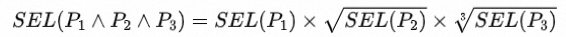This algorithm does not affect benchmark-generated datasets (such as TPCH) but can effectively reduce estimation errors in datasets based on real-world data.

## 4. Evaluation of Optimization Effect

We have tested the performance of IMCI on/off query optimization on the TPCH 1TB dataset, as shown in the following figure:It can be seen that for multi-table joins (such as Q8 and Q9), after IMCI query optimization is enabled, the execution efficiency is significantly increased because a better query plan is selected. In the workload of user scenarios, the query effect of enabling and disabling the query optimization function is compared, as shown in the following figure:

Before query optimization is enabled: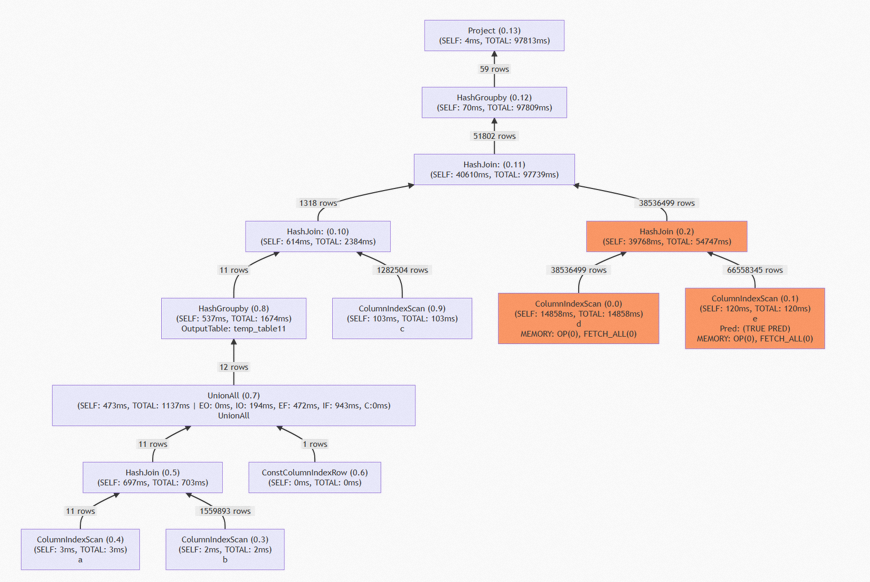If query optimization is not enabled, the join is performed based on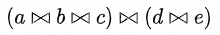It can be seen that the join (orange part) of tables d and e in the figure process a large amount of data, and more than 60% of the whole query is used to process these large table joins.

After query optimization is enabled: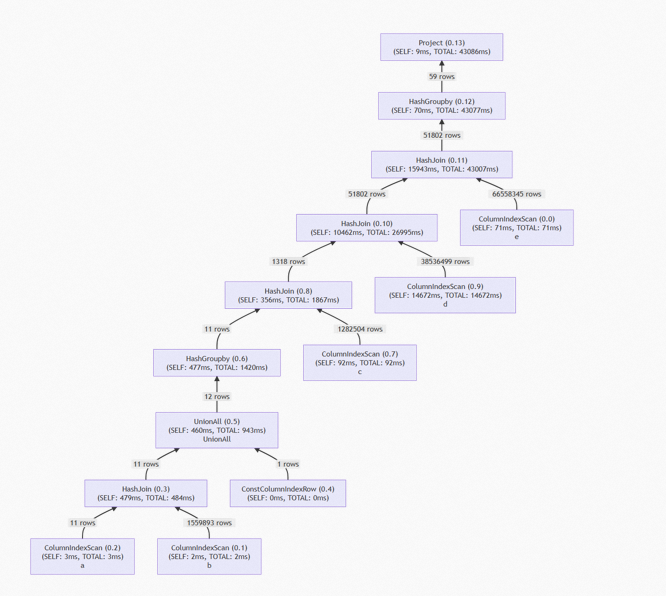The join order becomes: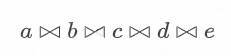The large table join of d and e is eliminated, and the execution time is reduced by more than 50%.

## 5. Problems to be Solved

### 5.1 More Rewriting Rules for Execution Plans and Better Heuristic Algorithm

In the process of solving join reorder, the join order obtained from the row-based optimizer is currently regarded as the result of the heuristic algorithm. As mentioned above, the execution plan may not be suitable for column execution models, so the subsequent IMCI will improve the heuristic algorithm when there are too many tables. The plan is to use the search space linearization technique in 2 to reduce search space and improve the possibility of finding a better plan on the premise of ensuring the quality of the plan.

On the other hand, IMCI doesn't have many rewrite rules, which are mainly used to deal with join reorder and a small number of rewrites for groupby. In the future, IMCI will introduce more rewrite rules for execution plans to expand search space and increase the possibility of finding better execution plans.

### 5.2 Execution Optimization of Hybrid Plans

IMCI has a limited ability to use row-based indexes. After the executor is combined with the determinant executor to execute SQL through hybrid execution plans, the optimizer needs to implement a large number of new functions, such as:

• New Implement Rules: The selection of algorithm selection steps is added, and new execution algorithms are needed (such as IndexScan, IndexJoin, and Streaming Groupby).
• Cardinality Estimation through Secondary Indexes: Compared with the statistical information obtained by sampling, indexes are equivalent to a histogram built by full table data. Therefore, compared with ordinary sampling, the status on secondary indexes is more accurate and comprehensive, which helps improve the accuracy of cardinality estimation.
• Accurate Cost Models: As secondary indexes are more likely to be out of the memory BufferPool compared with column index, in terms of cost models, we need to estimate the amount of I/O that may be generated by queries and compute I/O cost to select a better query plan more accurately.

### 5.3 More Accurate Statistics

At present, the statistical information of IMCI is mainly constructed based on sampling. Since the sampling ratio is more or less an error, we can consider drawing on the idea of stream computing to increase the accuracy of statistical information. When data is inserted, a sufficiently lightweight stream system is used to compute statistical information.

As such, it can be considered that secondary indexes in 5.2 are a heavy stream system, and the commonly used HyperLogLog is a good example of a light stream system. Umbra database uses HyperLogLog to maintain the number of distinct values in each column in real-time. HyperLogLog (a data structure that supports streaming approximate computing) is collectively called Sketch. Currently, there are many related studies. For example, we can find many encapsulated sketches in Apache datasketches, some of which can be used to replace histograms for range query estimation and distinct value estimation.

## References

 G. Graefe et al., “The Cascades Framework for Query Optimization," IEEE Data Eng. Bull., vol. 18, no. 3, pp. 337-348, 1995.

 Thomas Neumann and Bernhard Radke. 2018. Adaptive Optimization of Very Large Join Queries. In Proceedings of the 2018 International Conference on Management of Data (SIGMOD '18). Association for Computing Machinery, New York, NY, USA, 677-692. https://doi.org/10.1145/3183713.3183733

 Surajit Chaudhuri, Rajeev Motwani, and Vivek Narasayya. 1998. Random sampling for histogram construction: how much is enough?SIGMOD Rec. 27, 2 (June 1998), 436-447. https://doi.org/10.1145/276305.276343

 Surajit Chaudhuri, Gautam Das, and Utkarsh Srivastava. 2004. Effective use of block-level sampling in statistics estimation. In Proceedings of the 2004 ACM SIGMOD international conference on Management of data (SIGMOD '04). Association for Computing Machinery, New York, NY, USA, 287-298. https://doi.org/10.1145/1007568.1007602

0 1 0
Share on

# Related Products

• ##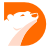PolarDB

Alibaba Cloud PolarDB is a cloud-native relational database service that decouples computing resources from storage resources

• ##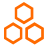PolarDB-X

Designed to address database challenges such as ultra-high concurrency, massive data storage, and large table performance bottlenecks.

• ##PolarDB Stack

A cloud-native database management platform that allows you to manage on-premises databases in the same way as in Alibaba Cloud.

• ##Database for FinTech Solution

Leverage cloud-native database solutions dedicated for FinTech.